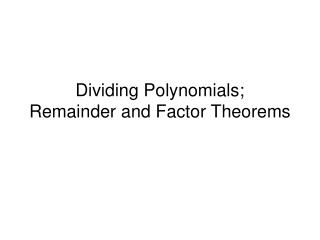DownloadDownload PresentationDividing Polynomials; Remainder and Factor Theorems

# Dividing Polynomials; Remainder and Factor Theorems

Télécharger la présentation## Dividing Polynomials; Remainder and Factor Theorems

- - - - - - - - - - - - - - - - - - - - - - - - - - - E N D - - - - - - - - - - - - - - - - - - - - - - - - - - -
##### Presentation Transcript

1. Dividing Polynomials;Remainder and Factor Theorems

2. Objectives • Use long division to divide polynomials. • Use synthetic division to divide polynomials. • Evaluate a polynomials using the Remainder Theorem. • Use the Factor Theorem to solve a polynomial equation.

3. How do you divide a polynomial by another polynomial? • Perform long division, as you do with numbers! Remember, division is repeated subtraction, so each time you have a new term, you must SUBTRACT it from the previous term. • Work from left to right, starting with the highest degree term. • Just as with numbers, there may be a remainder left. The divisor may not go into the dividend evenly.

4. Example • Divide using long division. State the quotient, q(x), and the remainder, r(x). (6x³ + 17x² + 27x + 20) (3x + 4)

5. Example • Divide using long division. State the quotient, q(x), and the remainder, r(x).

6. Remainders can be useful! • The remainder theorem states: If the polynomial f(x) is divided by (x – c), then the remainder is f(c). • If you can quickly divide, this provides a nice alternative to evaluating f(c).

7. Quick method of dividing polynomials Used when the divisor is of the form x – c Last column is always the remainder Synthetic Division

8. Example • Divide using synthetic division.

9. Example • Divide using synthetic division.

10. Factor Theorem • f(x) is a polynomial, therefore f(c) = 0 if and only if x – c is a factor of f(x). • Or in other words, • If f(c) = 0, then x – c is a factor of f(x). • If x – c is a factor of f(x), then f(c) = 0. • If we know a factor, we know a zero! • If we know a zero, we know a factor!

11. Zero of Polynomials If f(x) is a polynomial and if c is a number such that f(c) = 0, then we say that c is a zero of f(x). The following are equivalent ways of saying the same thing. • c is a zero of f(x) • x – c is a factor of f(x)

12. Example • Use the Remainder Theorem to find the indicated function value.